# Mean State

Period Mean (original grids) [W/m2]
Model Period Mean (intersection) [W/m2]
Model Period Mean (complement) [W/m2]
Benchmark Period Mean (intersection) [W/m2]
Benchmark Period Mean (complement) [W/m2]
Bias [W/m2]
RMSE [W/m2]
Phase Shift [months]
Bias Score 
RMSE Score 
Seasonal Cycle Score 
Spatial Distribution Score 
Interannual Variability Score 
Overall Score 
Benchmark [-] 401.
CLM4 [-] 397. 398. 0.00 400. 415. -1.37 9.58 1.81 0.50 0.39 0.73 0.91 0.65 0.60
CLM4.5 [-] 397. 398. 0.00 400. 415. -1.37 9.58 1.81 0.50 0.39 0.73 0.91 0.65 0.60
CLM5 [-] 397. 398. 0.00 400. 415. -1.37 9.58 1.81 0.50 0.39 0.73 0.91 0.65 0.60
Period Mean (original grids) [W/m2]
Model Period Mean (intersection) [W/m2]
Model Period Mean (complement) [W/m2]
Benchmark Period Mean (intersection) [W/m2]
Benchmark Period Mean (complement) [W/m2]
Bias [W/m2]
RMSE [W/m2]
Phase Shift [months]
Bias Score 
RMSE Score 
Seasonal Cycle Score 
Spatial Distribution Score 
Interannual Variability Score 
Overall Score 
Benchmark [-] 391.
CLM4 [-] 386. 386. 0.00 391. 404. -4.87 12.4 0.491 0.73 0.66 0.95 0.91 0.71 0.77
CLM4.5 [-] 386. 386. 0.00 391. 404. -4.87 12.4 0.491 0.73 0.66 0.95 0.91 0.71 0.77
CLM5 [-] 386. 386. 0.00 391. 404. -4.87 12.4 0.491 0.73 0.66 0.95 0.91 0.71 0.77
Period Mean (original grids) [W/m2]
Model Period Mean (intersection) [W/m2]
Model Period Mean (complement) [W/m2]
Benchmark Period Mean (intersection) [W/m2]
Benchmark Period Mean (complement) [W/m2]
Bias [W/m2]
RMSE [W/m2]
Phase Shift [months]
Bias Score 
RMSE Score 
Seasonal Cycle Score 
Spatial Distribution Score 
Interannual Variability Score 
Overall Score 
Benchmark [-] 367.
CLM4 [-] 361. 361. 0.00 366. 384. -2.96 12.2 0.935 0.71 0.62 0.88 0.99 0.75 0.76
CLM4.5 [-] 361. 361. 0.00 366. 384. -2.96 12.2 0.935 0.71 0.62 0.88 0.99 0.75 0.76
CLM5 [-] 361. 361. 0.00 366. 384. -2.96 12.2 0.935 0.71 0.62 0.88 0.99 0.75 0.76
Period Mean (original grids) [W/m2]
Model Period Mean (intersection) [W/m2]
Model Period Mean (complement) [W/m2]
Benchmark Period Mean (intersection) [W/m2]
Benchmark Period Mean (complement) [W/m2]
Bias [W/m2]
RMSE [W/m2]
Phase Shift [months]
Bias Score 
RMSE Score 
Seasonal Cycle Score 
Spatial Distribution Score 
Interannual Variability Score 
Overall Score 
Benchmark [-] 243.
CLM4 [-] 237. 237. 0.00 242. 256. -2.60 13.7 0.123 0.92 0.80 0.99 0.99 0.89 0.90
CLM4.5 [-] 237. 237. 0.00 242. 256. -2.60 13.7 0.123 0.92 0.80 0.99 0.99 0.89 0.90
CLM5 [-] 237. 237. 0.00 242. 256. -2.60 13.7 0.123 0.92 0.80 0.99 0.99 0.89 0.90
Period Mean (original grids) [W/m2]
Model Period Mean (intersection) [W/m2]
Model Period Mean (complement) [W/m2]
Benchmark Period Mean (intersection) [W/m2]
Benchmark Period Mean (complement) [W/m2]
Bias [W/m2]
RMSE [W/m2]
Phase Shift [months]
Bias Score 
RMSE Score 
Seasonal Cycle Score 
Spatial Distribution Score 
Interannual Variability Score 
Overall Score 
Benchmark [-] 411.
CLM4 [-] 411. 411. 0.00 409. 416. 3.75 11.6 1.88 0.37 0.37 0.71 0.57 0.75 0.52
CLM4.5 [-] 411. 411. 0.00 409. 416. 3.75 11.6 1.88 0.37 0.37 0.71 0.57 0.75 0.52
CLM5 [-] 411. 411. 0.00 409. 416. 3.75 11.6 1.88 0.37 0.37 0.71 0.57 0.75 0.52
Period Mean (original grids) [W/m2]
Model Period Mean (intersection) [W/m2]
Model Period Mean (complement) [W/m2]
Benchmark Period Mean (intersection) [W/m2]
Benchmark Period Mean (complement) [W/m2]
Bias [W/m2]
RMSE [W/m2]
Phase Shift [months]
Bias Score 
RMSE Score 
Seasonal Cycle Score 
Spatial Distribution Score 
Interannual Variability Score 
Overall Score 
Benchmark [-] 268.
CLM4 [-] 265. 264. 0.00 267. 280. -2.61 10.9 0.0463 0.92 0.85 1.0 0.97 0.86 0.91
CLM4.5 [-] 265. 264. 0.00 267. 280. -2.61 10.9 0.0463 0.92 0.85 1.0 0.97 0.86 0.91
CLM5 [-] 265. 264. 0.00 267. 280. -2.61 10.9 0.0463 0.92 0.85 1.0 0.97 0.86 0.91
Period Mean (original grids) [W/m2]
Model Period Mean (intersection) [W/m2]
Model Period Mean (complement) [W/m2]
Benchmark Period Mean (intersection) [W/m2]
Benchmark Period Mean (complement) [W/m2]
Bias [W/m2]
RMSE [W/m2]
Phase Shift [months]
Bias Score 
RMSE Score 
Seasonal Cycle Score 
Spatial Distribution Score 
Interannual Variability Score 
Overall Score 
Benchmark [-] 297.
CLM4 [-] 293. 292. 0.00 296. 341. -0.587 14.2 0.144 0.84 0.81 0.99 0.99 0.79 0.87
CLM4.5 [-] 293. 292. 0.00 296. 341. -0.587 14.2 0.144 0.84 0.81 0.99 0.99 0.79 0.87
CLM5 [-] 293. 292. 0.00 296. 341. -0.587 14.2 0.144 0.84 0.81 0.99 0.99 0.79 0.87
Period Mean (original grids) [W/m2]
Model Period Mean (intersection) [W/m2]
Model Period Mean (complement) [W/m2]
Benchmark Period Mean (intersection) [W/m2]
Benchmark Period Mean (complement) [W/m2]
Bias [W/m2]
RMSE [W/m2]
Phase Shift [months]
Bias Score 
RMSE Score 
Seasonal Cycle Score 
Spatial Distribution Score 
Interannual Variability Score 
Overall Score 
Benchmark [-] 387.
CLM4 [-] 374. 375. 0.00 385. 415. -5.63 15.4 0.408 0.72 0.71 0.96 1.0 0.80 0.82
CLM4.5 [-] 374. 375. 0.00 385. 415. -5.63 15.4 0.408 0.72 0.71 0.96 1.0 0.80 0.82
CLM5 [-] 374. 375. 0.00 385. 415. -5.63 15.4 0.408 0.72 0.71 0.96 1.0 0.80 0.82
Period Mean (original grids) [W/m2]
Model Period Mean (intersection) [W/m2]
Model Period Mean (complement) [W/m2]
Benchmark Period Mean (intersection) [W/m2]
Benchmark Period Mean (complement) [W/m2]
Bias [W/m2]
RMSE [W/m2]
Phase Shift [months]
Bias Score 
RMSE Score 
Seasonal Cycle Score 
Spatial Distribution Score 
Interannual Variability Score 
Overall Score 
Benchmark [-] 249.
CLM4 [-] 243. 244. 0.00 248. 253. -4.14 13.6 0.0505 0.92 0.80 1.0 0.98 0.91 0.90
CLM4.5 [-] 243. 244. 0.00 248. 253. -4.14 13.6 0.0505 0.92 0.80 1.0 0.98 0.91 0.90
CLM5 [-] 243. 244. 0.00 248. 253. -4.14 13.6 0.0505 0.92 0.80 1.0 0.98 0.91 0.90
Period Mean (original grids) [W/m2]
Model Period Mean (intersection) [W/m2]
Model Period Mean (complement) [W/m2]
Benchmark Period Mean (intersection) [W/m2]
Benchmark Period Mean (complement) [W/m2]
Bias [W/m2]
RMSE [W/m2]
Phase Shift [months]
Bias Score 
RMSE Score 
Seasonal Cycle Score 
Spatial Distribution Score 
Interannual Variability Score 
Overall Score 
Benchmark [-] 353.
CLM4 [-] 351. 351. 0.00 352. 359. -0.968 9.75 0.299 0.86 0.76 0.98 0.99 0.87 0.87
CLM4.5 [-] 351. 351. 0.00 352. 359. -0.968 9.75 0.299 0.86 0.76 0.98 0.99 0.87 0.87
CLM5 [-] 351. 351. 0.00 352. 359. -0.968 9.75 0.299 0.86 0.76 0.98 0.99 0.87 0.87
Period Mean (original grids) [W/m2]
Model Period Mean (intersection) [W/m2]
Model Period Mean (complement) [W/m2]
Benchmark Period Mean (intersection) [W/m2]
Benchmark Period Mean (complement) [W/m2]
Bias [W/m2]
RMSE [W/m2]
Phase Shift [months]
Bias Score 
RMSE Score 
Seasonal Cycle Score 
Spatial Distribution Score 
Interannual Variability Score 
Overall Score 
Benchmark [-] 314.
CLM4 [-] 306. 306. 0.00 312. 329. -3.25 11.6 0.223 0.81 0.75 0.98 1.0 0.70 0.83
CLM4.5 [-] 306. 306. 0.00 312. 329. -3.25 11.6 0.223 0.81 0.75 0.98 1.0 0.70 0.83
CLM5 [-] 306. 306. 0.00 312. 329. -3.25 11.6 0.223 0.81 0.75 0.98 1.0 0.70 0.83
Period Mean (original grids) [W/m2]
Model Period Mean (intersection) [W/m2]
Model Period Mean (complement) [W/m2]
Benchmark Period Mean (intersection) [W/m2]
Benchmark Period Mean (complement) [W/m2]
Bias [W/m2]
RMSE [W/m2]
Phase Shift [months]
Bias Score 
RMSE Score 
Seasonal Cycle Score 
Spatial Distribution Score 
Interannual Variability Score 
Overall Score 
Benchmark [-] 345.
CLM4 [-] 309. 309. 0.00 315. 360. -2.77 13.1 0.509 0.76 0.69 0.94 1.0 0.80 0.81
CLM4.5 [-] 309. 309. 0.00 315. 360. -2.77 13.1 0.509 0.76 0.69 0.94 1.0 0.80 0.81
CLM5 [-] 310. 310. 0.00 315. 360. -2.77 13.1 0.509 0.76 0.69 0.94 1.0 0.80 0.81
Period Mean (original grids) [W/m2]
Model Period Mean (intersection) [W/m2]
Model Period Mean (complement) [W/m2]
Benchmark Period Mean (intersection) [W/m2]
Benchmark Period Mean (complement) [W/m2]
Bias [W/m2]
RMSE [W/m2]
Phase Shift [months]
Bias Score 
RMSE Score 
Seasonal Cycle Score 
Spatial Distribution Score 
Interannual Variability Score 
Overall Score 
Benchmark [-] 350.
CLM4 [-] 344. 344. 0.00 349. 373. -3.78 14.4 0.431 0.82 0.73 0.96 0.99 0.79 0.84
CLM4.5 [-] 344. 344. 0.00 349. 373. -3.78 14.4 0.431 0.82 0.73 0.96 0.99 0.79 0.84
CLM5 [-] 344. 344. 0.00 349. 373. -3.78 14.4 0.431 0.82 0.73 0.96 0.99 0.79 0.84
Period Mean (original grids) [W/m2]
Model Period Mean (intersection) [W/m2]
Model Period Mean (complement) [W/m2]
Benchmark Period Mean (intersection) [W/m2]
Benchmark Period Mean (complement) [W/m2]
Bias [W/m2]
RMSE [W/m2]
Phase Shift [months]
Bias Score 
RMSE Score 
Seasonal Cycle Score 
Spatial Distribution Score 
Interannual Variability Score 
Overall Score 
Benchmark [-] 248.
CLM4 [-] 243. 243. 0.00 247. 258. -4.19 13.4 0.126 0.90 0.79 0.99 1.0 0.90 0.90
CLM4.5 [-] 243. 243. 0.00 247. 258. -4.19 13.4 0.126 0.90 0.79 0.99 1.0 0.90 0.90
CLM5 [-] 243. 243. 0.00 247. 258. -4.19 13.4 0.126 0.90 0.79 0.99 1.0 0.90 0.90
Period Mean (original grids) [W/m2]
Model Period Mean (intersection) [W/m2]
Model Period Mean (complement) [W/m2]
Benchmark Period Mean (intersection) [W/m2]
Benchmark Period Mean (complement) [W/m2]
Bias [W/m2]
RMSE [W/m2]
Phase Shift [months]
Bias Score 
RMSE Score 
Seasonal Cycle Score 
Spatial Distribution Score 
Interannual Variability Score 
Overall Score 
Benchmark [-] 376.
CLM4 [-] 363. 363. 0.00 371. 402. -1.23 14.5 0.704 0.64 0.68 0.91 0.99 0.78 0.78
CLM4.5 [-] 363. 363. 0.00 371. 402. -1.23 14.5 0.704 0.64 0.68 0.91 0.99 0.78 0.78
CLM5 [-] 363. 363. 0.00 371. 402. -1.23 14.5 0.704 0.64 0.68 0.91 0.99 0.78 0.78
Period Mean (original grids) [W/m2]
Model Period Mean (intersection) [W/m2]
Model Period Mean (complement) [W/m2]
Benchmark Period Mean (intersection) [W/m2]
Benchmark Period Mean (complement) [W/m2]
Bias [W/m2]
RMSE [W/m2]
Phase Shift [months]
Bias Score 
RMSE Score 
Seasonal Cycle Score 
Spatial Distribution Score 
Interannual Variability Score 
Overall Score 
Benchmark [-] 335.
CLM4 [-] 328. 328. 0.00 333. 372. -2.37 9.64 0.122 0.92 0.83 0.99 1.0 0.81 0.90
CLM4.5 [-] 328. 328. 0.00 333. 372. -2.37 9.64 0.122 0.92 0.83 0.99 1.0 0.81 0.90
CLM5 [-] 328. 328. 0.00 333. 372. -2.37 9.64 0.122 0.92 0.83 0.99 1.0 0.81 0.90
Period Mean (original grids) [W/m2]
Model Period Mean (intersection) [W/m2]
Model Period Mean (complement) [W/m2]
Benchmark Period Mean (intersection) [W/m2]
Benchmark Period Mean (complement) [W/m2]
Bias [W/m2]
RMSE [W/m2]
Phase Shift [months]
Bias Score 
RMSE Score 
Seasonal Cycle Score 
Spatial Distribution Score 
Interannual Variability Score 
Overall Score 
Benchmark [-] 278.
CLM4 [-] 275. 275. 0.00 277. 303. -0.971 9.62 0.0673 0.91 0.82 1.0 0.99 0.86 0.90
CLM4.5 [-] 275. 275. 0.00 277. 303. -0.971 9.62 0.0673 0.91 0.82 1.0 0.99 0.86 0.90
CLM5 [-] 275. 275. 0.00 277. 303. -0.971 9.62 0.0673 0.91 0.82 1.0 0.99 0.86 0.90
Period Mean (original grids) [W/m2]
Model Period Mean (intersection) [W/m2]
Model Period Mean (complement) [W/m2]
Benchmark Period Mean (intersection) [W/m2]
Benchmark Period Mean (complement) [W/m2]
Bias [W/m2]
RMSE [W/m2]
Phase Shift [months]
Bias Score 
RMSE Score 
Seasonal Cycle Score 
Spatial Distribution Score 
Interannual Variability Score 
Overall Score 
Benchmark [-] 298.
CLM4 [-] 293. 294. 0.00 298. 325. -3.00 12.5 0.124 0.83 0.76 0.99 0.99 0.74 0.85
CLM4.5 [-] 293. 294. 0.00 298. 325. -3.00 12.5 0.124 0.83 0.76 0.99 0.99 0.74 0.85
CLM5 [-] 293. 294. 0.00 298. 325. -3.00 12.5 0.124 0.83 0.76 0.99 0.99 0.74 0.85
Period Mean (original grids) [W/m2]
Model Period Mean (intersection) [W/m2]
Model Period Mean (complement) [W/m2]
Benchmark Period Mean (intersection) [W/m2]
Benchmark Period Mean (complement) [W/m2]
Bias [W/m2]
RMSE [W/m2]
Phase Shift [months]
Bias Score 
RMSE Score 
Seasonal Cycle Score 
Spatial Distribution Score 
Interannual Variability Score 
Overall Score 
Benchmark [-] 410.
CLM4 [-] 406. 406. 0.00 410. 415. -2.98 10.8 1.26 0.55 0.47 0.84 0.98 0.77 0.68
CLM4.5 [-] 406. 406. 0.00 410. 415. -2.98 10.8 1.26 0.55 0.47 0.84 0.98 0.77 0.68
CLM5 [-] 406. 406. 0.00 410. 415. -2.98 10.8 1.26 0.55 0.47 0.84 0.98 0.77 0.68
Period Mean (original grids) [W/m2]
Model Period Mean (intersection) [W/m2]
Model Period Mean (complement) [W/m2]
Benchmark Period Mean (intersection) [W/m2]
Benchmark Period Mean (complement) [W/m2]
Bias [W/m2]
RMSE [W/m2]
Phase Shift [months]
Bias Score 
RMSE Score 
Seasonal Cycle Score 
Spatial Distribution Score 
Interannual Variability Score 
Overall Score 
Benchmark [-] 379.
CLM4 [-] 377. 376. 0.00 380. 374. -2.98 11.2 0.682 0.77 0.65 0.94 0.99 0.84 0.81
CLM4.5 [-] 377. 376. 0.00 380. 374. -2.98 11.2 0.682 0.77 0.65 0.94 0.99 0.84 0.81
CLM5 [-] 377. 376. 0.00 380. 374. -2.98 11.2 0.682 0.77 0.65 0.94 0.99 0.84 0.81
Period Mean (original grids) [W/m2]
Model Period Mean (intersection) [W/m2]
Model Period Mean (complement) [W/m2]
Benchmark Period Mean (intersection) [W/m2]
Benchmark Period Mean (complement) [W/m2]
Bias [W/m2]
RMSE [W/m2]
Phase Shift [months]
Bias Score 
RMSE Score 
Seasonal Cycle Score 
Spatial Distribution Score 
Interannual Variability Score 
Overall Score 
Benchmark [-] 303.
CLM4 [-] 301. 301. 0.00 298. 346. 6.58 22.1 0.556 0.48 0.53 0.95 0.93 0.75 0.70
CLM4.5 [-] 301. 301. 0.00 298. 346. 6.58 22.1 0.556 0.48 0.53 0.95 0.93 0.75 0.70
CLM5 [-] 301. 301. 0.00 298. 346. 6.58 22.1 0.556 0.48 0.53 0.95 0.93 0.75 0.70

# Temporally integrated period mean

BENCHMARK MEAN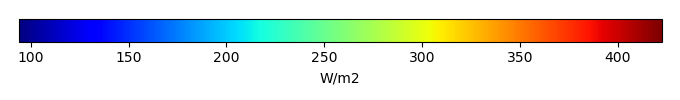MODEL MEANBIAS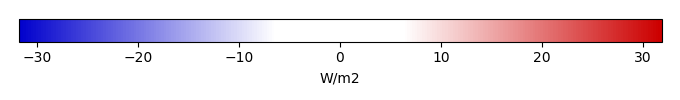BIAS SCORERMSE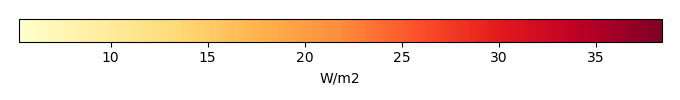RMSE SCOREBENCHMARK INTERANNUAL VARIABILITY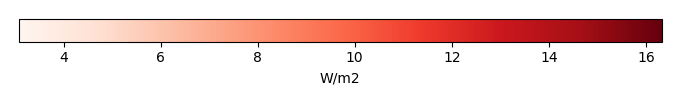MODEL INTERANNUAL VARIABILITYINTERANNUAL VARIABILITY SCOREBENCHMARK MAX MONTHMODEL MAX MONTHDIFFERENCE IN MAX MONTH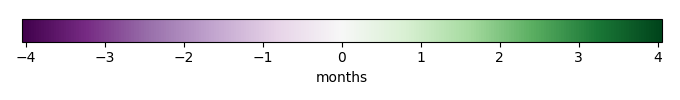SEASONAL CYCLE SCORESPATIAL TAYLOR DIAGRAMMODEL COLORS# Spatially integrated regional mean

MODEL COLORSREGIONAL MEANANNUAL CYCLEMONTHLY ANOMALYANNUAL CYCLE# All Models

BenchmarkCLM4CLM4.5CLM5# Data Information

creation_date: Thu May 8 23:18:00 PDT 2014

source_file: This product is generated from monthly 1 degree CERES EBAF Radiation observations